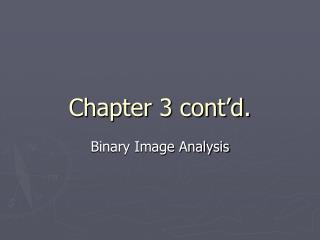# Chapter 3 cont’d. - PowerPoint PPT PresentationDownload PresentationChapter 3 cont’d.

Chapter 3 cont’d.Download Presentation## Chapter 3 cont’d.

- - - - - - - - - - - - - - - - - - - - - - - - - - - E N D - - - - - - - - - - - - - - - - - - - - - - - - - - -
##### Presentation Transcript

1. Chapter 3 cont’d. Binary Image Analysis

2. Binary image morphology (nonlinear image processing)

3. closing

4. Definition: closing simply dilation followed by erosion when –B = B comp((comp(A) opened by B))

5. Application to medical images

6. Application to Gear inspection (missing teeth)

7. Extracting shape primitives

8. Morphological filter properties • translation invariance (E,D,O,C)

9. Morphological filter properties • increasing (E,D,O,C)

10. Morphological filter properties • commutative (D) • associative (D) This property can lead to a more efficient implementation. How?

11. Morphological filter properties • antiextensive (O)

12. Morphological filter properties • extensive (C)

13. Morphological filter properties • idempotent (O,C) • “Idempotence is the property of certain operations in mathematics and computer science, that they can be applied multiple times without changing the result beyond the initial application.” - wikipedia

14. Morphological filter properties • The origin is important for E and D, but not O and C.

15. Boundaries

16. Boundary detection • Let B be a disk centered at the origin. Then we can detect 3 types of boundaries: 1. External boundary 2. Internal boundary 3. Both (morphological gradient)

17. Boundary example

18. More examples input dilation external erosion morphological gradient internal

19. More examples

20. Dilation & distance maps/transforms Dilation can be used to create distance maps. Di is the set of all pixels at distance i from object. The operators denote the ith and i-1th applications of dilation.

21. Conditional dilation

22. Conditional dilation • (Two somewhat conflicting) problems: • Dilation may merge separate objects. • Erosion may remove parts of an otherwise complete object that we are trying to target.

23. Conditional dilation (Dougherty et al. method) addressing problem #1 (i.e., dilation may merge separate objects) • If B contains the origin, expands A without bound. Recall dilation: • So we define conditional dilation: - the conditional dilation of A by B relative to C

24. Conditional dilation (Dougherty) cont’d. • But the previous definition may cause dilation to join disconnected objects so another form may be used:

25. Conditional dilation (Dougherty) example

26. Conditional dilation (Shapiro and Stockman) addressing problem #2 • Recall problem #2: “We want the entire component(s), not just what remains of them after erosion.” • “Given an original binary image B, a processed image C (e.g., C=B(-)V for some structuring element V), and a structuring element S, let The conditional dilation of C by S w.r.t. B is defined by where the index m is the smallest index satisfying Cm = Cm-1.”

27. Example of Shapiro and Stockman conditional dilation Note: C is (typically) a processed version of B. From previous slide: “Given an original binary image B, a processed image C, and a structuring element S, let The conditional dilation of C by S w.r.t. B is defined by where the index m is the smallest index satisfying Cm = Cm-1.” Basically, we grow (dilate) object seeds in C until we completely recover the object in B corresponding to those seeds. S S

28. Conditional dilation example Below are the input image (left), C, and the result of its dilation by an euclidean disk of diameter 7 (right), C0<i<m. Below are the conditional image (left), B, and the resultant image (right), Cm.

29. Skeletonization

30. Skeletonization • a common thinning procedure • Medial Axis Transform (MAT) • Connected sets can have disconnected skeletons!

31. Skeletonization

32. Skeletonization Definition: Given a Euclidean (continuous) binary image, consider a point within an object. Next, consider that point to be the center of the largest disk centered at that point s.t. the edge of the disk touches the border of the object. Two possibilities: 1. This disk is contained in a larger disk (centered somewhere else) or 2. This disk is NOT contained in a larger disk. If (2) holds, the disk is maximal and the center is on the medial axis of the object.

33. Skeletonization

34. Skeletonization A and B are maximal; C is not.

35. Skeletonization

36. Skeletonizationexample Note: I’m not sure why these three aren’t exactly the same!

37. Skeletonization example

38. Skeletonization • Define skeletal subset Skel(S; n) to be the set of all pixels x in S s.t. x is the center of a maximal disk nB (the n-fold dilation) where • n=0,1,… and • (n in nB is, in effect, the size.) • Define skeleton to be the union of all skeletal subsets:

39. Morphological operations on gray images • Believe or not, these operations can be (have been) extended to gray image data! • This ends our discussion of binary morphological operations. • References: • E. Dougherty, “An Introduction to Morphological Image Processing,” SPIE Press, 1992. • E. R. Dougherty and J. Astola, “An Introduction to Nonlinear Image Processing,” SPIE Press, 1994. • C.R. Giardina and E.R. Dougherty, “Morphological Methods in Image and Signal Processing,” Prentice Hall, 1988. • L.G. Shapiro and G.C. Stockman, “Computer Vision,” Prentice Hall, 2001.